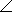# Online Electrical Engineering Test - Electrical Engineering Test - RandomLoading Test...

Instruction:

• This is a FREE online test. DO NOT pay money to anyone to attend this test.
• Total number of questions : 20.
• Time alloted : 30 minutes.
• Each question carry 1 mark, no negative marks.
• DO NOT refresh the page.
• All the best :-).

1.

When a solenoid is activated, the force that moves the plunger is

A.
 an electromagnetic fieldB.
 a permanent magnetic fieldC.
 varying voltageD.
 a steady current2.

What is the reluctance of a material that has a length of 0.07 m, a cross-sectional area of 0.014 m2, and a permeability of 4,500Wb/At × m?

A.
 1111 At/WbB.
 111 At/WbC.
 11 At/WbD.
 1 At/Wb3.

How many primary volts must be applied to a transformer with a turns ratio of 0.1 to obtain a secondary voltage of 9 V?

A.
 9 VB.
 90 VC.
 900 VD.
 0.9 VExplanation:

We know that, V2/V1 = N2/N1.

Given V2 = 9v, N2/N1 = 0.1

Therefore, 9v/V1 = 0.1

V1 = 9v/0.1 = 90v.

For more detail: Step Down Transformer - Circuit with Simulation.

4.

The primary winding of a transformer has 110 V ac across it. What is the secondary voltage if the turns ratio is 8?

A.
 8.8 VB.
 88 VC.
 880 VD.
 8,800 VExplanation:

V2/V1 = N2/N1

Turns ratio = N2/N1 = 8.

V2/110 = 8

V2 = 8 x 110

V2 = 880v.

5.

The turns ratio required to match an 80source to a 320load is

A.
 80B.
 20C.
 4D.
 26.

To increase the current in a series RL circuit, the frequency

A.
 should be increasedB.
 should be decreasedC.
 should be constantD.
 cannot be determined without values7.

When the frequency is decreased, the impedance of a parallel RL circuit

A.
 increasesB.
 decreasesC.
 remains constantD.
 is not a factor8.

Norton's theorem gives

A.
 an equivalent current source in parallel with an equivalent impedanceB.
 an equivalent current source in series with an equivalent impedanceC.
 an equivalent voltage source in parallel with an equivalent impedanceD.
 an equivalent voltage source in series with an equivalent impedance9.

Find the current in R2 of the given circuit, using the superposition theorem.A.
 16.7 mAB.
 33.3 mAC.
 50 mAD.
 16.6 mA10.

An autotransformer is one in which the primary and secondary are automatically coupled.

A.
 TrueB.
 False11.

In an ac circuit, power to the load peaks at the frequency at which the load impedance is the complex conjugate of the output impedance.

A.
 TrueB.
 False12.

The number 4,500,000 can be expressed as

A.
 4,500 × 106B.
 4.5 × 106C.
 4.5 × 10-3D.
 either 4,500 × 104 or 4.5 × 10613.

The number of millivolts in 0.06 kilovolts is

A.
 600 VB.
 6,000 mVC.
 60,000 mVD.
 600,000 mV14.

Polyphase is characterized by two or more sinusoidal voltages, each having a different phase angle.

A.
 TrueB.
 False15.

How much current is produced by a voltage of 18 kV across a 15 kresistance?

A.
 1.2 AB.
 12 AC.
 120 mAD.
 12 mA16.

The unit of electrical charge is the

A.
 coulombB.
 jouleC.
 voltD.
 watt17.

When a 1.6 kresistor and a 120resistor are connected in parallel, the total resistance is

A.
 greater than 1.6 kB.
 greater than 120but less than 1.6 kC.
 less than 120but greater than 100D.
 less than 10018.

The complex number 4055° is equivalent to

A.
 55 + j55B.
 40 + j40C.
 45.88 + j65.52D.
 22.94 + j32.7619.

Using the current-divider formula, you can find the current in any branch of a series-parallel circuit.

A.
 TrueB.
 False20.

The branch current method uses

A.
 Kirchhoff's voltage and current lawsB.
 Thevenin's theorem and Ohm's lawC.
 Kirchhoff's current law and Ohm's lawD.
 the superposition theorem and Thevenin's theorem